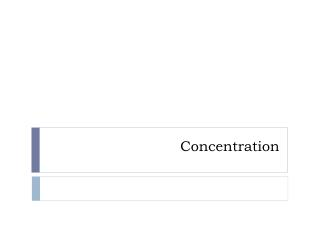DownloadDownload PresentationConcentration

# Concentration

Télécharger la présentation## Concentration

- - - - - - - - - - - - - - - - - - - - - - - - - - - E N D - - - - - - - - - - - - - - - - - - - - - - - - - - -
##### Presentation Transcript

1. Concentration

2. Molarity • Moles of solute per liter of solution

3. Ex. 1 • What is the molarity of a solution that was prepared by dissolving 11.5g of solid sodium hydroxide in 1.50L of water?

4. Ex. 2 • Calculate the molarity of a solution prepared by dissolving 1.56g of gaseous HCl in enough water to make 26.8 mL of solution.

5. Examples • Calculate the number of moles of chloride ions in 1.75L of .0010 M zinc chloride. • Typical blood serum is about 0.14M NaCl. What volume of blood contains 1.0mg of NaCl? • To analyze the alcohol content of a certain wine, a chemist needs 1.00 L of an aqueous potassium dichromate solution. How much solid must be weighed out to make this solution?

6. Dilution • When water is added to a concentrated solution in order to achieve the desired molarity (molarity)(volume) = (molarity)(volume)

7. Ex. • What volume of 16M sulfuric acid must be used to prepare 1.5L of a .10 M solution?